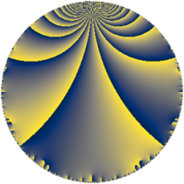# Properties

 Label 117.4.gLevel $117$ Weight $4$ Character orbit 117.g Rep. character $\chi_{117}(55,\cdot)$ Character field $\Q(\zeta_{3})$ Dimension $34$ Newform subspaces $6$ Sturm bound $56$ Trace bound $2$

# Related objects

## Defining parameters

 Level: $$N$$ $$=$$ $$117 = 3^{2} \cdot 13$$ Weight: $$k$$ $$=$$ $$4$$ Character orbit: $$[\chi]$$ $$=$$ 117.g (of order $$3$$ and degree $$2$$) Character conductor: $$\operatorname{cond}(\chi)$$ $$=$$ $$13$$ Character field: $$\Q(\zeta_{3})$$ Newform subspaces: $$6$$ Sturm bound: $$56$$ Trace bound: $$2$$ Distinguishing $$T_p$$: $$2$$

## Dimensions

The following table gives the dimensions of various subspaces of $$M_{4}(117, [\chi])$$.

Total New Old
Modular forms 92 38 54
Cusp forms 76 34 42
Eisenstein series 16 4 12

## Trace form

 $$34 q + 3 q^{2} - 69 q^{4} + 12 q^{5} + 9 q^{7} - 66 q^{8} + O(q^{10})$$ $$34 q + 3 q^{2} - 69 q^{4} + 12 q^{5} + 9 q^{7} - 66 q^{8} - 3 q^{10} + 33 q^{11} - 16 q^{13} - 180 q^{14} - 289 q^{16} + 81 q^{17} - 241 q^{19} - 213 q^{20} - 170 q^{22} + 159 q^{23} + 830 q^{25} + 87 q^{26} + 284 q^{28} + 207 q^{29} + 300 q^{31} + 297 q^{32} - 1382 q^{34} + 270 q^{35} - 733 q^{37} - 1188 q^{38} + 1622 q^{40} - 627 q^{41} - 63 q^{43} - 1128 q^{44} + 694 q^{46} + 1644 q^{47} - 278 q^{49} + 2046 q^{50} + 346 q^{52} - 2004 q^{53} - 1046 q^{55} + 1800 q^{56} - 1525 q^{58} - 189 q^{59} - 465 q^{61} + 1488 q^{62} + 6290 q^{64} + 978 q^{65} + 1817 q^{67} + 807 q^{68} + 2344 q^{70} - 171 q^{71} - 2964 q^{73} + 1137 q^{74} - 5072 q^{76} - 4806 q^{77} + 1680 q^{79} - 4149 q^{80} - 4017 q^{82} - 3624 q^{83} + 2452 q^{85} + 1428 q^{86} - 4608 q^{88} + 2967 q^{89} - 2451 q^{91} - 2088 q^{92} + 3508 q^{94} + 4608 q^{95} + 2225 q^{97} - 1167 q^{98} + O(q^{100})$$

## Decomposition of $$S_{4}^{\mathrm{new}}(117, [\chi])$$ into newform subspaces

Label Dim $A$ Field CM Traces $q$-expansion
$a_{2}$ $a_{3}$ $a_{5}$ $a_{7}$
117.4.g.a $2$ $6.903$ $$\Q(\sqrt{-3})$$ None $$-1$$ $$0$$ $$-14$$ $$10$$ $$q+(-1+\zeta_{6})q^{2}+7\zeta_{6}q^{4}-7q^{5}+10\zeta_{6}q^{7}+\cdots$$
117.4.g.b $2$ $6.903$ $$\Q(\sqrt{-3})$$ None $$3$$ $$0$$ $$18$$ $$-2$$ $$q+(3-3\zeta_{6})q^{2}-\zeta_{6}q^{4}+9q^{5}-2\zeta_{6}q^{7}+\cdots$$
117.4.g.c $2$ $6.903$ $$\Q(\sqrt{-3})$$ None $$4$$ $$0$$ $$-34$$ $$-20$$ $$q+(4-4\zeta_{6})q^{2}-8\zeta_{6}q^{4}-17q^{5}-20\zeta_{6}q^{7}+\cdots$$
117.4.g.d $4$ $6.903$ $$\Q(\sqrt{-3}, \sqrt{17})$$ None $$-5$$ $$0$$ $$30$$ $$-15$$ $$q+(-2+\beta _{1}+2\beta _{2}+\beta _{3})q^{2}-5\beta _{1}q^{4}+\cdots$$
117.4.g.e $8$ $6.903$ $$\mathbb{Q}[x]/(x^{8} - \cdots)$$ None $$2$$ $$0$$ $$12$$ $$14$$ $$q+\beta _{1}q^{2}+(-1+\beta _{1}+5\beta _{2}-\beta _{3}+\beta _{5}+\cdots)q^{4}+\cdots$$
117.4.g.f $16$ $6.903$ $$\mathbb{Q}[x]/(x^{16} + \cdots)$$ None $$0$$ $$0$$ $$0$$ $$22$$ $$q+\beta _{1}q^{2}+(-5-5\beta _{2}+\beta _{3}+\beta _{6})q^{4}+\cdots$$

## Decomposition of $$S_{4}^{\mathrm{old}}(117, [\chi])$$ into lower level spaces

$$S_{4}^{\mathrm{old}}(117, [\chi]) \cong$$ $$S_{4}^{\mathrm{new}}(13, [\chi])$$$$^{\oplus 3}$$$$\oplus$$$$S_{4}^{\mathrm{new}}(39, [\chi])$$$$^{\oplus 2}$$